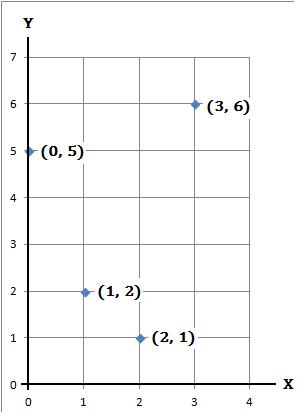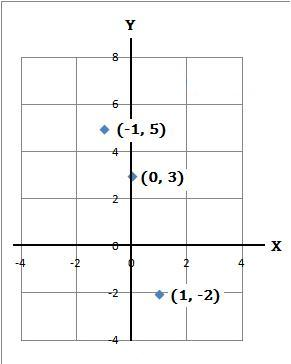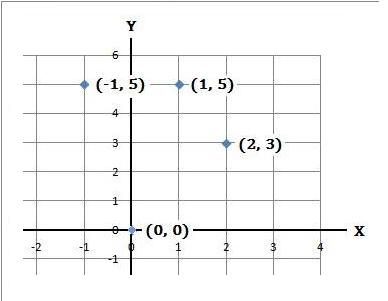#### 1.   Plot the four coordinates (0, 5), (1, 2), (2, 1) and (3, 6)

(a) Make a table of the coordinates

 x 0 1 2 3 y 5 2 1 6

(b) Plot the coordinates#### 2.   Plot the three coordinates (−1, 5), (0, 3) and (1, −2)

(a) Make a table of the coordinates

 x −1 0 1 y 5 3 −2

(b) Plot the coordinates#### 3.   Plot the four coordinates (−1, 5), (0, 0), (1, 5) and (2, 3)

(a) Make a table of the coordinates

 x −1 0 1 2 y 5 0 5 3

(b) Plot the coordinatesback to: